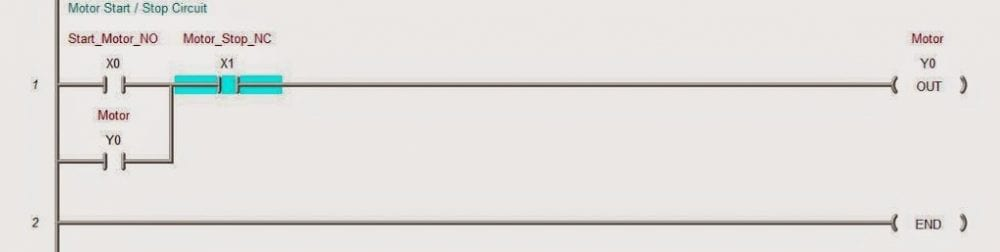# Here’s a Quick Way to Understand PLC Inputs and Outputs

The term I/O means Input/Output. I/O can come in two different types; Discrete or Analog Most people starting out leaning about programmable logic controls (PLC) are taught all about discrete input and outputs. Data is received from devices such as push-buttons, limit-switches etc. and devices are turned on such as motor contactor, lights, etc. Discrete input and output bits are either on or off. (1 or 0) The following program will show a motor control circuit stop start. Motor off:

Motor on:

Analog inputs Common input variables for analog are temperature, flow, pressure, etc. They are converted to an electrical signal into a PLC analog input. Standard electrical signals are 0 – 20 mA, 4 – 20 mA, 0 – 10 volts DC, -10 – 10 volts DC. Note: It is recommended that a 4 – 20 mA signal is best. If voltage is required, a resistor can be added to get a voltage input. Analog outputs Common output variables for analog are speed, flow, pressure, etc. They are converted from a word in the PLC to the output of the analog. The range of signal is then outputted to the device to control the position, rate, etc. Standard electrical signals to the device are 4 – 20 mA, 0 – 10 volts DC, -10 – 10 volts DC. Both Analog Inputs and Outputs use words to determine the signal going to or from the device. Example: 4 – 20 mA current Input – 8 bit resolution 4 mA = 000000002 = 0016 20 mA = 11111111= FF16 Example: 4 – 20 mA current Output – 8 bit resolution 0016 = 000000002 = 4 mA FF16 = 111111112 =20 mA For a review of numbering systems, follow the link below: What everyone should know about PLC numbering systems

Let me know if you have any questions or need further information.
Thank you,
Garry

If you’re like most of my readers, you’re committed to learning about technology. Numbering systems used in PLC’s are not difficult to learn and understand. We will walk through the numbering systems used in PLCs. This includes Bits, Decimal, Hexadecimal, ASCII and Floating Point.

To get this free article, subscribe to my free email newsletter.

Use the information to inform other people how numbering systems work. Sign up now.